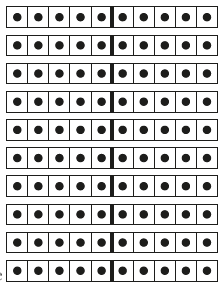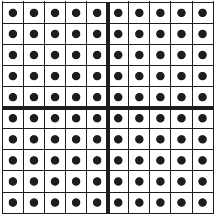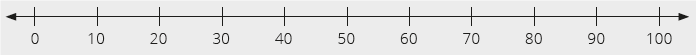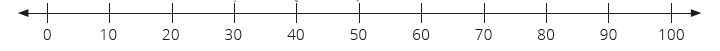# Lesson Notes By Weeks and Term - Primary 2

Number sequences up to 100

TERM: 1st Term

WEEK: 3

CLASS: Primary 2

AGE: 7 years

DURATION: 5 periods of 40 minutes each

DATE:

SUBJECT: Mathematics

TOPIC: Number sequences up to 100

SPECIFIC OBJECTIVES: At the end of the lesson, the pupils should be able to

1. Counting forwards and backwards in 5s and 10s up to 100.
2. Count forwards and backwards in fives and tens from any number – they

use this to investigate ways of building up and breaking down numbers.

INSTRUCTIONAL TECHNIQUES: Explanation, question and answer, demonstration, practical

INSTRUCTIONAL MATERIALS: Printed tens, bottle tops and place value table per learner, 1 printed hundred (teacher), a board game for each pair of learners, dice.

PERIOD 1: Number sequences up to 100

 PRESENTATION TEACHER’S ACTIVITY PUPIL’S ACTIVITY STEP 1MENTAL MATHS The teacher begins the lesson with some mental calculationsThe teacher uses the mental Maths time to revise the use of the printed tens and hundreds.Ten of theseAnd 1 hundred• Revises with the learners the structure of printed tens and hundreds. Ten tens makes1 hundred.• Allows the learners time to match the ten printed tens onto the 1 hundred square.• Asks the learners to count with you forwards and backwards from 10, 20…..100 as you point to the separate tens and also the columns/rows in the printed hundred.• Asks a learner to come to the board to show you 50 – either using the printed hundred or the printed tens.• Now asks the learners what is 10 less than 50? (40)- What is 10 more than 50? (60)• Repeats these questions with 30, 80 and 60. Pupils respond and participate STEP 2CONCEPTDEVELOPMENT The teacher• Shows the learners ten fingers, and ask them how many fingers there are. (10)Close your hands, and ask: How many fingers do I have? (10) How many of them can you see? (0).• Asks two learners to come to the front of the class. Ask both learners to lift up both hands.• Asks: How many fingers are there? (20)• Asks a third learner to come to the front of the class. Ask all three learners to lift up theirhands. Ask, How many fingers are there now? (30)• Repeats the exercise with a fourth learner lifting their hands in the air. Ask, how manyfingers are there now? (40)• Asks the learners to count all the fingers again, counting in 10s. (10, 20, 30, 40) CLASS ACTIVITYThe teacher• Draw a fives number line from 50 to 100 on the board.• Asks the learners to count in tens from 50 to 100, pointing at each multiple of 10 on thenumber line.• As they count, circles the multiples of ten on the number line. (50, 60, 70, 80, 90, 100)• Discusses why you have circled these numbers.• Discusses the fives that have been labelled on this number line but that there are also other numbers that fall in between the labelled numbers.• Asks: What other numbers lie between 55 and 60? (Learners may answer 56, 57, 58, 59)• Asks the same question but for other number intervals.• Points to 55 and ask the learners what is 5 more than 55? (60) What is 5 lessthan 55? (50)• Uses the number line to assist you showing the learners 5 more and 5 less each time.• Repeats 5 more/less with 70, 85 and 95. ACTVITY IIThe teacher• Writes the multiples of 10 between 0 and 100 on the board in a random order.• Asks the learners to write the numbers from 10 to 40 on the board from the smallest to the biggest number. (10, 20, 30, 40)• Now asks the class to write them in order from the biggest to the smallest number (40, 30, 20, 10).• Asks the learners to write the numbers from 50 to 100 in order from the smallest to the biggest number on the board. (50, 60, 70, 80, 90, 100)• Discusses how they sorted the numbers (they have written them going up inmultiples of 10)• Asks them to use the same numbers and order them from the biggest to the smallestnumber. (100, 90, 80, 70, 60, 50).• Explains to them that this is called counting back in 10s – from 100 to 50.• Discusses how they sorted the numbers (they have written them going down inmultiples of 10) Pupils pay attention and participate STEP 3CLASS-WORK 1 If 2 children stand in front of the class, how many fingers will you see?2 If 3 children stand in front of the class, how many fingers will you see?3 Continue the pattern patterns of 10:a 10, 20, 30, __, __.b 30, 40, 50, __, __.c 100, 90, 80, __, __.d 70, 60, 50, __, __.4 Complete the table:5 more than: 5 less than:a 10 is __       b 35 is __       c 50 is __       d 85 is __       e 5 is__f 15 is __g 30 is __h 100 is ___ Pupils attempt their class work STEP 4HOME-WORK 1 Complete the pattern of 10: 10, 20, 30, ____, ____, ____, ____, ___, ____, 100.2 Fill in the missing numbers: 5, 10, ____, _____, _____, 30, _____, 40.3 Continue the pattern:a 60, 50, _____, ____, _____.b 90, 80, _____, ____, _____, 40, _____, _____.c 50, 45, _____, 35 , _____, _____, 20. The pupils writes it in their homework book STEP 5SUMMARY The teacher summarizes by reminding the pupils that in this lesson they learnt how to count in 5s and 10s to 100. We have revised what more and less mean. She marks their class works, makes corrections where necessary and commends them positively

PERIOD 2: Number sequences up to 100

 PRESENTATION TEACHER’S ACTIVITY PUPIL’S ACTIVITY STEP 1MENTAL MATHS The teacher begins the lesson with some mental calculationsWhat is the next number1 5, 10, ...       2 70, 60, ...      3 34, 35, ...      4 99, 98, ...      5 25, 30, ...     6 10, 20, ...7 65, 70, ...8 80, 75, ...9 28, 29, ...10 82, 81, ... Pupils respond and participate STEP 2CONCEPTDEVELOPMENT The teacher• Asks 12 learners to stand in front of the class.• Asks the class how many legs the first learner has. (2)• Asks the learners the following questions and remind them to count in twos:- How many legs do the first and the second learners have altogether? (4)- How many legs do the first, second and third learners have altogether? (6)- How many legs do the first, second, third and fourth learners have altogether? (8)• Repeats until you have reached the twelfth learner.• Asks all the learners to count all the legs again. (2, 4, 6, 8, 10, 12, 14, 16, 18, 20, 22, 24)• Discusses the counting pattern you have just shown – the pattern of 2s – counting goes upin multiples of 2. CLASS ACTIVITYThe teacher• Writes the following numbers on the board: 20, 54, 66,7,37 and 45.• Arranges them in order from smallest to biggest. (7, 20, 37, 45, 54 and 66)• Gives the learners a 100 board.• Using the numbers above, (7, 20, 37, 45, 54 and 66) add 7 more to each of the numbers.(14, 27,44,52,61 and 73). Let learners use the 100 board as an aid.• Using the numbers above. (7, 20, 37, 45, 54 and 66) go to 7 less than each of the numbers. (0, 13, 30, 38, 47 and 59) Let learners use the 100 board as an aid. ACTIVITY IIThe teacher• In pairs, lets the learners play the snake and ladder game below. While they play thegame you can circulate and speak to them about the moves they are making using ofmathematical language.Pupils pay attention and participate STEP 3CLASS-WORK 1 Write these numbers from the greatest to the smallest: 53, 59, 54, 56.2 Give two numbers that are greater than 56, but not bigger than 60.3 Give two numbers that are smaller than 54, but not smaller than 51.4 Write the number name for the number that is greater than 54, but smaller than 56.5 What is the value of the 3 in 73?6 What is the value of the 7 in 75?7 Complete the following:a 7 less than 80 is ___b 7 more than 57 is ___ Pupils attempt their class work STEP 4HOME-WORK 1 Complete the pattern: 68, 69, __, 71, __, __.2 What is 7 less than 75?3 What is two more than 71?4 Thelma has 60 marbles. He finds 7 more. How many does he now have altogether? The pupils writes it in their homework book STEP 5SUMMARY The teacher summarizes by reminding the pupils that in today’s lesson we counted in 2s, compared numbers and added and subtracted 7 from various numbers She marks their class works, makes corrections where necessary and commends them positively

PERIOD 3: Building up and breaking down numbers

 PRESENTATION TEACHER’S ACTIVITY PUPIL’S ACTIVITY STEP 1MENTAL MATHS The teacher begins the lesson with some mental calculationsWhat is 2 less than1 4   2 2    3 10  4 12  5 206 14  7 228 169 2410 18 Pupils respond and participate STEP 2CONCEPTDEVELOPMENT The teacher• Draws a fives number line from 0 to 50 on the board.• Asks the learners to count in fives from 5 to 50.• As they count, circles the multiples of five on the number line. (5, 10, 15, 20, 25, 30, 35, 40, 45, 50)• Discusses why you have circled these numbers. (We are counting in 5s.)• Makes sure that learners understand and can identify numbers that are between given numbers, e.g. 2 is between 0 and 5, 34 is between 30 and 35, and so on. CLASS ACTIVITYThe teacher• Gives each pair a 100 board.• Revises with the learners counting in 10s to 100 using the board as an aid.• Asks each pair to show each other the number 40 on the number board. (4 groups of 10)• Asks: What is 10 more than 40? They should show each other the answer using the number board. (50)• Asks: What is 10 less than 40? They should show you the answer using the number board. (30)• Repeats 10 more and 10 less using 30, 50 and 90.• If learners can answer without using the number board this is good. Encourages them to draw on their number knowledge and not be bound to point on the number board. CLASS ACTIVITYThe teacherCopies the following number line onto the board• Asks learners to count in tens from 0 to 100, pointing at each multiple of 10 on thenumber line.• Asks: What do you notice that is the same on the 100 board and the number line?(The learners should notice the patterns of 10 are on both.)• Writes the following questions on the board:10 + 10 =60 + 10 =30 + 10 =• Asks the learners to solve these questions using the number line. Show the learners how to add 10 and 10 by moving forwards along the number line. Repeat with 60 + 10 = and30 + 10 =• Uses the number line to show the learners how we get to the answer.• Writes the following questions on the board:50 – 10 =30 – 10 =10 – 10 =• Using the number line point to 50. Shows the learners how we subtract 10 from 50 bymoving backwards along the number line. Repeats with 30 – 10 = and 10 – 10 = Pupils pay attention and participate STEP 3CLASS-WORK 1 Circle the following numbers on the number line 10, 30, 40, 70, 90.2 Solve the following:a 30 + 10 = __b 50 + 10 = __c 90 + 10 = __d 90 – 10 = __e 60 – 10 = __f 40 – 10 = __ 3 Complete the following:a 90 + ____ = 100b 50 + ____ = 100c 40 + ____ = 100d 30 + ____ = 100e 20 + ____ = 100 Pupils attempt their class work STEP 4HOME-WORK Complete the following:a 60 + 10 = ____b 40 + 10 = ____c 70 – 10 = ____d 30 – 10 = ____e 20 + ____ = 100 The pupils writes it in their homework book STEP 5SUMMARY The teacher summarizes by reminding the pupils that in today’s lesson we counted and compared numbers in 5s and 10s up to 100. We have also made the number 100 using multiples of 10. She marks their class works, makes corrections where necessary and commends them positively

PERIOD 4: Assessment

PRESENTATION

TEACHER’S ACTIVITY

PUPIL’S ACTIVITY

STEP 1

ORAL

ASSESSMENTS

The teacher asks questions on mental Maths treated so far

Pupils respond and participate

STEP 2

DISCUSSION

The teacher discusses all the methods used by some learners in the oral assessments(some of the questions are solved on the board by the learners) and addresses any misconceptions that may have risen

Pupils pay attention and participate

STEP 3

WRITTEN ASSESSMENTS

1 Show the number 26 by drawing the dots on the ten frames.

2 Circle the biggest number, and make a cross over the smallest number.

 48 21 19 38 14 12 44

3 Show 30 + 20 using the number line below:4 Fill in the missing numbers: 30, 40, __, __, __, 80, __, 100.

5 Write these numbers from the greatest to the smallest: 93, 99, 94, and 96

6 Give two numbers that are greater than 97, but not bigger than 100.

7 Give two numbers that are smaller than 74, but not smaller than 71.

8 What is the value of the 6 in 56?

9 What is the value of the 8 in 85?

10 Complete the table:

 7 less than 7 more than 10 _____ 20 _____ 20 _____ 90 _____ 30 _____ 40 _____

Pupils attempt their class work

STEP 4

SUMMARY

The teacher marks the written assessments, corrects were necessary and commends the pupils

PERIOD 5: Weekly Test/consolidations

TEACHER’S ACTIVITY: The teacher revises all the concepts treated from period 1-4 and gives the pupils follow through exercises, quiz and tests. She marks the exercises, makes corrections and commends the pupils positively.

PUPIL’S ACTIVITY: The pupils work on the worksheets and exercises give by the teacher individually

CONSOLIDATION

1 Complete:

a 40 + __ = 100

b 30 + __ = 100

c __ + 50 = 100

2 Complete:

a Which number is two less than 14? __

b Which number is two more than 26? __

c Which number is five more than 70? __

d Which number is ten more than 90? __

e Which number is five less than 55? __

f Which number is ten less than 100? __

3 3 more than 30 is ____.

4 Fill in the missing numbers: 30, 40, __, __, __, 80, __, 100.

5 Complete:

a 6 groups of 10 is ___

b 36 has ___groups of 10 and___ ones

c 48 has ___groups of 10 and ____ones

6 Complete the pattern: 40, 50, __, 70, __, __.

7 What is 3 less than 30? __

8 What is 5 more than 75? __

9 7 groups of 10 is ___.